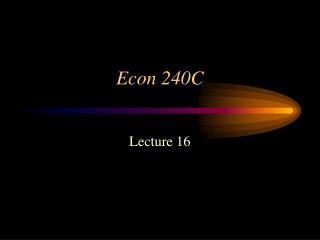DownloadDownload PresentationEcon 240C

# Econ 240C

Télécharger la présentation## Econ 240C

- - - - - - - - - - - - - - - - - - - - - - - - - - - E N D - - - - - - - - - - - - - - - - - - - - - - - - - - -
##### Presentation Transcript

1. Econ 240C Lecture 16

2. Part I. VAR • Does the Federal Funds Rate Affect Capacity Utilization?

3. The Federal Funds Rate is one of the principal monetary instruments of the Federal Reserve • Does it affect the economy in “real terms”, as measured by capacity utilization for total industry?

4. Preliminary Analysis • The Time Series, Monthly, January 1967 through April 2008

5. Capacity Utilization Total Industry: Jan. 1967- April 2008

6. Identification of TCU • Trace • Histogram • Correlogram • Unit root test • Conclusion: probably evolutionary

7. Identification of FFR • Trace • Histogram • Correlogram • Unit root test • Conclusion: unit root

8. Pre-whiten both

9. Changes in FFR & Capacity Utilization

10. Contemporaneous Correlation

11. Dynamics: Cross-correlation Two-Way Causality?

12. In Levels Too much structure in each hides the relationship between them

13. In differences

14. Granger Causality: Four Lags

15. Granger Causality: two lags

16. Granger Causality: Twelve lags

17. Estimate VAR

18. Estimation of VAR

19. Specification • Same number of lags in both equations • Use liklihood ratio tests to compare 12 lags versus 24 lags for example

20. Estimation Results • OLS Estimation • each series is positively autocorrelated • lags 1, 18 and 24 for dtcu • lags 1, 2, 4, 7, 8, 9, 13, 16 for dffr • each series depends on the other • dtcu on dffr: negatively at lags 10, 12, 17, 21 • dffr on dtcu: positively at lags 1, 2, 9, 24 and negatively at lag 12

21. Correlogram of DFFR

22. Correlogram of DTCU

23. Interpretation • We need help • Rely on assumptions

24. What If • What if there were a pure shock to dtcu • as in the primitive VAR, a shock that only affects dtcu immediately

25. Primitive VAR (tcu Notation) dtcu(t) =1 + 1 dffr(t) + 11 dtcu(t-1) + 12 dffr(t-1) + 1 x(t) + edtcu (t) (2) dffr(t) = 2 + 2 dtcu(t) + 21 dtcu(t-1) + 22 dffr(t-1) + 2 x(t) + edffr (t)

26. Primitive VAR (capu notation)

27. The Logic of What If • A shock, edffr , to dffr affects dffr immediately, but if dcapu depends contemporaneously on dffr, then this shock will affect it immediately too • so assume b1 iszero, then dcapu depends only on its own shock, edcapu , first period • But we are not dealing with the primitive, but have substituted out for the contemporaneous terms • Consequently, the errors are no longer pure but have to be assumed pure

28. DTCU shock DFFR

29. Standard VAR • dcapu(t) = (a1 + b1 a2)/(1- b1 b2) +[ (g11+ b1 g21)/(1- b1 b2)] dcapu(t-1) + [ (g12+ b1 g22)/(1- b1 b2)] dffr(t-1) + [(d1+ b1 d2 )/(1- b1 b2)] x(t) + (edcapu(t) + b1 edffr(t))/(1- b1 b2) • But if we assume b1 =0, • thendcapu(t) = a1 +g11 dcapu(t-1) + g12 dffr(t-1) + d1 x(t) + edcapu(t) +

30. Note that dffr still depends on both shocks • dffr(t) = (b2 a1 + a2)/(1- b1 b2) +[(b2 g11+ g21)/(1- b1 b2)] dcapu(t-1) + [ (b2 g12+ g22)/(1- b1 b2)] dffr(t-1) + [(b2 d1+ d2 )/(1- b1 b2)] x(t) + (b2edcapu(t) + edffr(t))/(1- b1 b2) • dffr(t) = (b2 a1 + a2)+[(b2 g11+ g21) dcapu(t-1) + (b2 g12+ g22) dffr(t-1) + (b2 d1+ d2 ) x(t) + (b2edcapu(t) + edffr(t))

31. Reality edtcu(t) DTCU shock DFFR edffr(t)

32. What If edtcu(t) DTCU shock DFFR edffr(t)